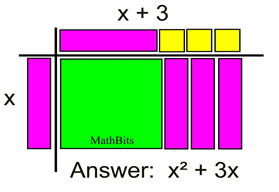Multiply Monomial by Polynomial MathBitsNotebook.com Terms of Use   Contact Person: Donna Roberts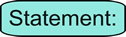When multiplying polynomials, each term from one polynomial must be multiplied by each term of the other polynomial.

Since each term in a polynomial is a monomial, multiplying polynomials becomes multiplying monomials.Multiply a Monomial by a Monomial: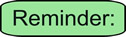When multiplying monomials, use the product rule for exponents.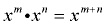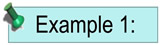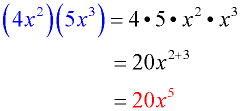The factors are regrouped, and then multiplied. Notice the product rule for exponents at work [when the bases are the same, add the exponents].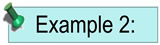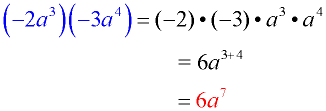Be careful of the signs associated with each term.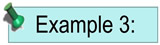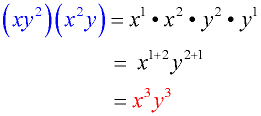If more than one variable is involved, group each variable separately. As with all mathematical procedures, as you become more confident in your skills, you may be able to process some of these steps mentally.Multiply a Monomial by a Polynomial:When multiplying a monomial by a polynomial, use the distributive property. The traditional statement of the distributive property shows a monomial times a binomial: a • (b + c) = a • b + a • c This statement can be expanded when more terms are involved:       a • (b + c + d + ... + n) = ab + ac + ad + ... + an.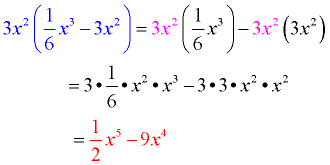(monomial x binomial) The 3x2 is distributed (multiplied) by each term in the binomial. The process then becomes multiplying a monomial times another monomial.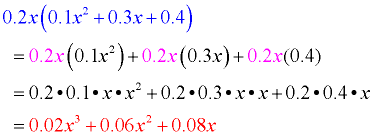(monomial x trinomial) Be careful to distribute the 0.2x times all three terms in the trinomial. Watch out for those decimal points!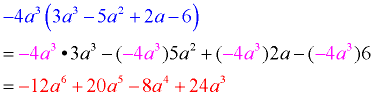(monomial x polynomial) Be sure to distribute -4a3 times all four terms. Be careful of the signs. Don't forget the negative sign in -4a3.Monomial Multiplication with Algebra Tiles:

 This set up of Algebra tiles gives you a "visual" demonstration of multiplying monomial x times binomial (x + 3). Key: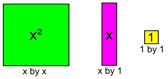See more about Algebra Tiles.